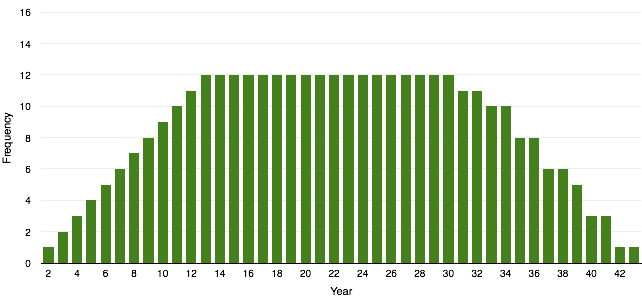# Math in Dates

As software engineer I see patterns. I noticed Wednesday the date was 7/8/15. This formed a simple math equation where the month plus the day equaled the two digit year: `m + d = yy`

So I wondered, what year would have the most dates that satisfy this equation?

Initially I thought this would be year 13 - since there are 12 months in a year the lowest possible sum is 13. However, this assumes two things.

First, it assumes there is a single maximum year. And after thinking about it more, I realized there can be multiple maximum years.

Second, it assumes there is only one equation per month satisfying a year. While this seems true, I was not the guy who could write equations on my dorm room window.

But I am the guy who can write a quick program to test these assumptions. The following is a script written in PHP which outputs the frequency of dates satisfying this equation per year:

``````__torchlight-block-[185276ba-15c5-4506-a782-449e0361d6c0]__
``````

This data forms the graph:As you can see it forms an even distribution between 13 and 30 (31 for leap years). So these years all tie with a maximum frequency of 12. Furthermore, since the maximum frequency is 12 and there are 12 months in the year the second assumption is true (for this equation).

While this does answer the main question, a few sub questions arose.

1. Is there a mathematical equation to represent this problem?
2. Is there a better programmatic solution? Better meaning performant or written in a language suited for such problems.